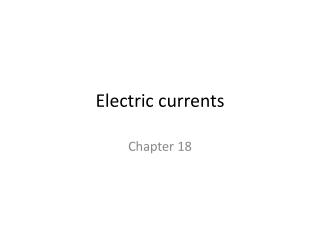Download PresentationElectric currents

Loading in 2 Seconds...

# Electric currents - PowerPoint PPT Presentation

Electric currents. Chapter 18. Electric Battery . Made of two or more plates or rods called electrodes. Electrodes are made of dissimilar metals Electrodes are immersed in an electrolyteI am the owner, or an agent authorized to act on behalf of the owner, of the copyrighted work described.
Download Presentation## Electric currents

An Image/Link below is provided (as is) to download presentation

Download Policy: Content on the Website is provided to you AS IS for your information and personal use and may not be sold / licensed / shared on other websites without getting consent from its author.While downloading, if for some reason you are not able to download a presentation, the publisher may have deleted the file from their server.

- - - - - - - - - - - - - - - - - - - - - - - - - - E N D - - - - - - - - - - - - - - - - - - - - - - - - - -
Presentation Transcript
1. Electric currents Chapter 18

2. Electric Battery • Made of two or more plates or rods called electrodes. • Electrodes are made of dissimilar metals • Electrodes are immersed in an electrolyte • The electrolyte will react with one metal in a way to dissolve positive ions into the solution thus becoming negatively charged. The other metal loses electrons to the electrolyte becoming positively charged and a potential difference (pressure difference) is created. • The electrodes in an electrolyte is a cell • Cells put together form a battery.

3. battery simulation

4. Electrical potential and Voltage • Electric potential (V)– the potential energy per unit charge • This is caused by the electrical force of repulsion. • Voltage- a difference in electrical potential • Considered electrical pressure • This is measured in volts • Flashlight batteries are 1.5V, Household outlets are 110V or 220V

5. Electric current • Current is the flow of charge through a circuit. • I = ΔQ/Δ t • Rate at which charge flows • Measured in Amperes • 1A = 1Coulomb/second • Electron Current • Negative electron flows from (-) to (+) circuit simulation • Conventional current • Positive charge flows from (+) to (-)

6. Ohm’s Law/ Resistance • For a current (flow of charge) to occur there must be a Voltage (electric pressure) difference. This can be produced by a battery. • How much current there is depends on how easily the charge can move through a circuit (resistance). • Current, Voltage, and resistance are all related by Ohm’s Law

7. RESISTORS • Electrical resistance is the opposition of the flow of electricity by some object or substance. • Resistors are used to control the flow of electricity in a circuit. • Every conductor has some resistance depending on the material. • resistance simulation • Resistance is measured in Ohms ( ) • 1  = 1V/1A • The bands on the resistor show how much resistance the resistor has • Uses for resistance • Electronic circuits (control flow of electricity) • Toaster, heating element, variable resistors • Lightbulbs

8. Ohm’s Law • Current, Voltage, and resistance are all related by Ohm’s Law • V = I *R • If you graph the relationship between voltage and current, the slope represents the resistance. • Current is consistent through a circuit but voltage changes.

9. Practice Ohm’s Law • A small flashlight bulb draws 300mA from its 1.5V battery. • What is the resistance of the bulb? • If the voltage dropped to 1.2V, how would the current change. • How many electrons would pass through the bulb in one second?

10. Resistivity • How resistive a material is • ρ = constant of proportionality/resistivity • Measured in Ohms/meter • Temperature dependent (α) • ρT = ρo[1+ α(T-To)] • Measure of the resistance in a wire. • Depends on? • Length, Cross-sectional area, resistivity • R= ρ(L/A)

11. Resistors in series and parallel (19.1) • When resistors are connected end to end they are said to be in series • Resistors can be bulbs, heating elements, …etc. • Voltage = I Req • For a series Circuit: Req = R1+R2+R3… • When resistors are on their own wire they are in parallel. • Voltage = I Req • For a series Circuit: 1/Req = 1/R1+1/R2+1/R3…

12. Practice • Find the equivalent resistance. R = 8 ohms Req= 4 ohms solution Req=11.1 ohms

13. Electric Power • Electric energy is useful because it can be transformed into mechanical energy to do work. • To find the power transformed by an electrical device: Power = energy transformed/time • Measured in Watts • Electric companies charge in kW*hrs

14. Practice • Your parents yell at you for leaving the lights on in the living room overnight. You want to find out how much you cost them. If there were 2 75Watt bulbs running for 7 hours and the electric company charges \$0.09 per kW*hr, how much do you owe them?